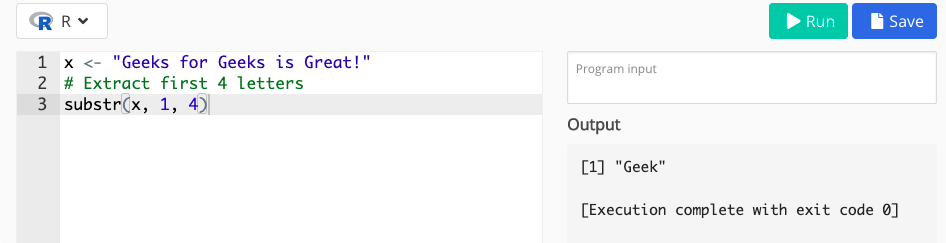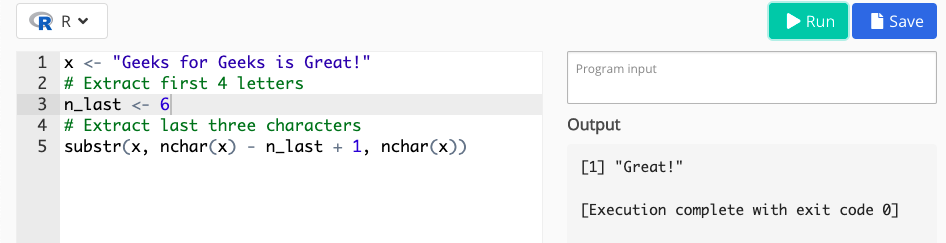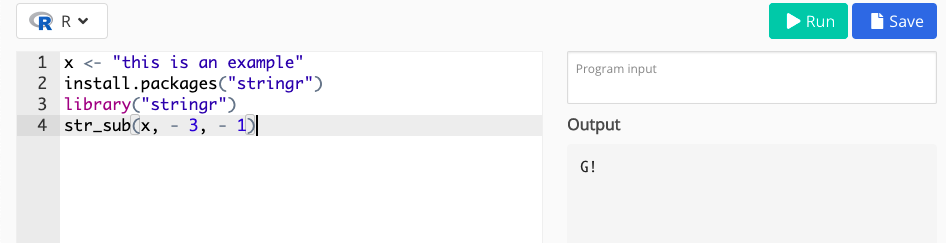# Extract First or Last n Characters from String in R

• Last Updated : 01 May, 2022

In this article, we will know how to extract the last and first char from a given string in the R programming language.

For the example purpose, “Geeks for Geeks is Great” is included in our example string. Let’s take a look at how we can extract the first and last n characters of this example string.

### Example 1: In R, extract the first n characters from a string:

We will learn how to obtain the first n characters of a string in the first example. We can use the substr function for this task:

In the below example, you can see that the substr function returned this to the RStudio console (i.e. the first three characters). To use the substr command to extract the first n characters, we are required to specify three values within the function:

• The string of characters (in our case x).
• We want to maintain the first character (in our case 1).
• The final character we wish to maintain is (in this specific example we extracted the first 3 values).

## R

 `x <- ``"Geeks for Geeks is Great!"`` ` `# Extract first 4 letters``substr``(x, 1, 4)`### Example 2: In Base R, extract the last n characters from a string:

Here, we will also utilize the substr function in the second example (as in Example 1). However, the code is becoming a little more complicated this time:

Based on the previous result, we can see that we have extracted the characters ple, i.e. the last three characters of our example string. To do so, we have to supply three inputs for the substr function once more:

• The string of characters (in our case x).
• We want to maintain the first character.
• To begin, we have to utilize the nchar function to determine the length of our string (i.e. nchar(x)).
• Then we needed to deduct the number of characters we wanted to extract from the length of our string (i.e. nchar(x) – n last).
• We had to add the value 1 to our computation since we needed to specify the starting character with the substr function (i.e. nchar(x) – n last + 1).
• The last character we wish to maintain (in this example, the last character of our string, nchar(x)).

This code works correctly, although it is admittedly more difficult than the code in Example 1. As a result, in the next example, I’ll show you a simpler way of extracting the final n characters of a string.

## R

 `x <- ``"Geeks for Geeks is Great!"`` ` `# Extract last six characters``n_last <- 6``substr``(x, ``nchar``(x) - n_last + 1, ``nchar``(x))`### Example 3: Using the stringr Package in R, extract the last n characters from a string:

The stringr R package makes it simple to get the last n characters of a string. First, let’s install and load the package.

Syntax to install and install the stringr package in the R console:

```install.packages("stringr")
library("stringr") ```

Str_sub() function: This will recycle all arguments to be the same length as the longest argument. If any arguments are of length 0, the output will be a zero length character vector

Syntax: str_sub(string, start = 1L, end = -1L)

Parameters:

• string: input character vector.
• start, end: Two integer vectors. start gives the position of the first character (defaults to first), end gives the position of the last (defaults to last character). Alternatively, pass a two-column matrix to start.Negative values count backwards from the last character.

In this example, we are simply calling the str_sub() function with the string and the first and the end position accordingly to get extract the last n characters from a string in the R programming language.

## R

 `x <- ``"Geeks for Geeks is Great!"`                  `install.packages``(``"stringr"``)               ``library``(``"stringr"``)     ``str_sub``(x, - 3, - 1)`My Personal Notes arrow_drop_up